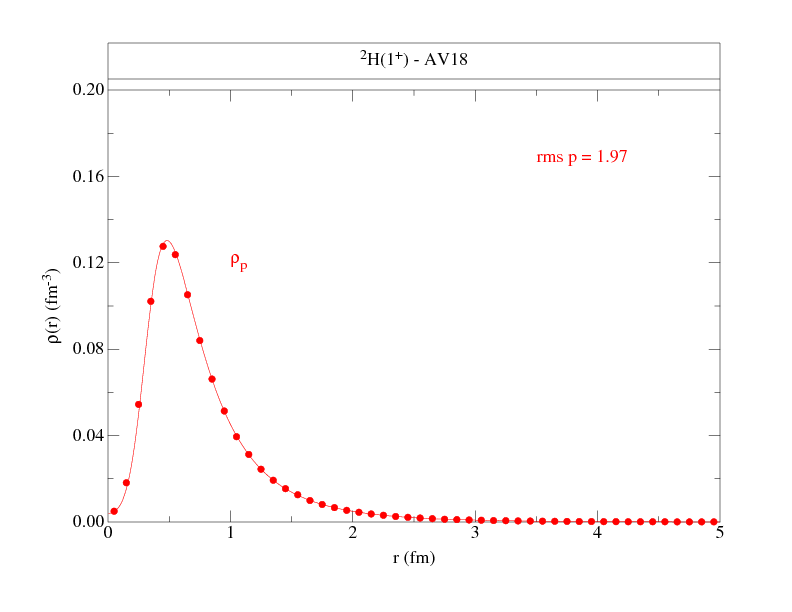# Single-Nucleon Densities

## Two-Nucleon DensitiesThis web page presents single-nucleon densities calculated for a variety of nuclei in the range A=2-12. Corresponding two-nucleon densities can be found here. These are from variational Monte Carlo calculations (VMC) using (unless otherwise noted) the Argonne v18 two-nucleon and Urbana X three-nucleon potentials (AV18+UX). (Urbana X is intermediate between the Urbana IX and Illinois-7 models; it has the form of UIX supplemented with a two-pion S-wave piece, while the strengths of its terms are taken from the IL7 model. It does NOT have the three-pion-ring term of IL7.)

--- NEW! ---
Results for some of the Norfolk NV2+3 potentials are now being added:
Piarulli, et al., Phys. Rev. C 94, 054007 (2016).
--- NEW! ---

These VMC wave functions are the starting trial functions for a number of recent Green's function Monte Carlo (GFMC) calculations:
Brida, et al., Phys. Rev. C 84, 024319 (2011);
McCutchan, et al., Phys. Rev. C 86, 024315 (2012);
Pastore, et al., Phys. Rev. C 87, 035503 (2013);
Pastore, et al., Phys. Rev. C 90, 024321 (2014).

More details of the wave function construction can be found in
Wiringa, Phys. Rev. C 43, 1585 (1991) for A=3,4;
Pudliner, et al., Phys. Rev. C 56, 1720 (1997) for A=6,7;
Wiringa, et al., Phys. Rev. C 62, 014001 (2000) for A=8;
Pieper, et al., Phys. Rev. C 70, 044310 (2002) for A=9,10.

The largest nuclei are evaluated using the cluster VMC (CVMC) method.

The CVMC method is described in
Pieper, et al., Phys. Rev. C 46, 1741 (1992) for A=16 with AV14+UVII
Lonardoni, et al., arXiv:1705.04337 for A=16,40 with AV18+UIX.

The results are generated as distributions for neutron spin-down, neutron spin-up, proton spin-down, and proton spin-up, for the M=J state. The densities are for the same wave functions used in generating the single-nucleon momentum distributions given here

Following are figures and files that tabulate the proton and neutron densities to give an overall view of their shapes. The normalization is chosen such that:

ANS = ∫ d3r ρNS(r)

where NS denotes proton or neutron, spin up or down, and ANS is the total number (out of A) nucleons with the given nucleon-spin projection. Where proton and neutron density distributions are the same, as in T=0 nuclei, we give only one set, and similarly, if spin-up and spin-down projections are the same, as in 0+ states, we give totals only.

 2H(1+) AV18 Figure 1 Figure 2 Table 2H(1+) NV2-Ia Figure 1 Figure 2 Table 2H(1+) NV2-Ib Figure 1 Figure 2 Table 2H(1+) NV2-IIa Figure 1 Figure 2 Table 2H(1+) NV2-IIb Figure 1 Figure 2 Table 3H(1/2+) Figure 1 Figure 2 Table 3He(1/2+) Figure 1 Figure 2 Table 4He(0+) Figure 1 Figure 2 Table 4He(0+) AV18 Figure 1 Figure 2 Table 5H(1/2+) Figure 1 Figure 2 Table 6He(0+) Figure 1 Figure 2 Table 6Li*(0+) Figure 1 Figure 2 Table 6Be(0+) Figure 1 Figure 2 Table 6Li(1+) Figure 1 Figure 2 Table 7He(3/2-) Figure 1 Figure 2 Table 7Li*(3/2-) Figure 1 Figure 2 Table 7Be*(3/2-) Figure 1 Figure 2 Table 7B(3/2-) Figure 1 Figure 2 Table 7Li(3/2-) Figure 1 Figure 2 Table 7Be(3/2-) Figure 1 Figure 2 Table 8He(0+) Figure 1 Figure 2 Table 8Li(2+) Figure 1 Figure 2 Table 8Be(0+) Figure 1 Figure 2 Table 8Be(0+) AV18+UIX Figure 1 Figure 2 Table 8B(2+) Figure 1 Figure 2 Table 8C(0+) Figure 1 Figure 2 Table 9Li(3/2-) Figure 1 Figure 2 Table 9Be(3/2-) Figure 1 Figure 2 Table 9C(3/2-) Figure 1 Figure 2 Table 10He(0+) Figure 1 Figure 2 Table 10Be(0+) Figure 1 Figure 2 Table 10B(3+) Figure 1 Figure 2 Table 10C(0+) Figure 1 Figure 2 Table 11Li(3/2-) Figure 1 Figure 2 Table 11B(3/2-) Figure 1 Figure 2 Table 11C(3/2-) Figure 1 Figure 2 Table 12Be(0+) Figure 1 Figure 2 Table 12B(1+) Figure 1 Figure 2 Table 12C(0+) Figure 1 Figure 2 Table 12C(0+) AV18+UIX Figure 1 Figure 2 Table 16O(0+) CVMC AV18+UIX Figure 1 Figure 2 Table 16O(0+) CVMC AV18 Figure 1 Figure 2 Table 40Ca(0+) CVMC AV18+UIX Figure 1 Figure 2 Table 40Ca(0+) CVMC AV18 Figure 1 Figure 2 Table

Robert B. Wiringa
Last update June 16, 2022